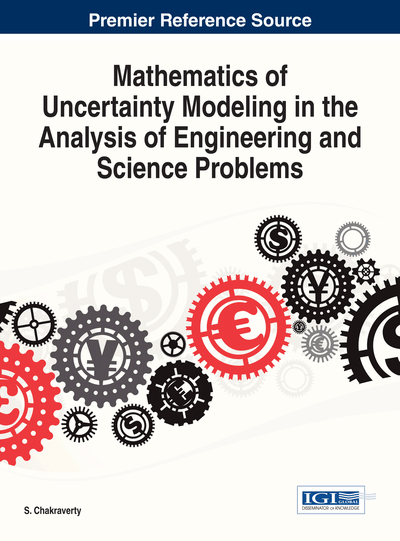# Modeling with Stochastic Fuzzy Differential Equations

Marek T. Malinowski
DOI: 10.4018/978-1-4666-4991-0.ch008
OnDemand:
(Individual Chapters)
Available
\$37.50
No Current Special Offers

## Abstract

In the chapter, the author considers an approach used in the studies of stochastic fuzzy differential equations. These equations are new mathematical tools for modeling uncertain dynamical systems. Some qualitative properties of their solutions such as existence and uniqueness are recalled, and stability properties are shown. Here, the solutions are continuous adapted fuzzy stochastic processes. The author considers some examples of applications of stochastic fuzzy differential equations in modeling real-world phenomena.
Chapter Preview
Top

## 1. Introduction

Applicability of ordinary differential equations in modeling dynamical systems is of a great importance. However, modeling with ordinary differential equations is suitable in case of a perfect knowledge about considered dynamical system. In particular, parameters of the system and its initial state have to be known precisely. They should also be described using the precise, single values (singletons) of a phase space. However, a typical feature of behavior of real dynamical systems is uncertainty, which contrasts with precision. The two most important kinds of uncertainties are randomness (stochastic uncertainty) and imprecision (sometimes called fuzziness, vagueness, ambiguity, and softness). For a long time, a usual way of describing uncertainty in modeling was a usage of stochastic models, which were based on probability theory and stochastic analysis. Stochastic models of dynamical systems in the form of random differential equations or Itô type stochastic differential equations do not include all kinds of uncertainties. They include stochastic uncertainty only. When data of the considered systems are imprecise due to lack of precision of measuring instruments there appears uncertainty which is not of stochastic type and stochastic models are no longer applicable. Uncertain information that is not stochastic in its nature is appropriately treated by fuzzy set theory (Zadeh, 1965; Negoita & Ralescu, 1975; Zimmermann, 1991). The linguistic variables e.g. “hot”, “about 5”, “greater than 100” can be modeled by fuzzy sets successfully.

The examinations of dynamic systems incorporating fuzziness resulted in extensive studies of fuzzy differential equations with solutions being fuzzy-valued functions. Here, the fuzziness is the only source of uncertainty. There are monographs dealing with fuzzy differential equations (Diamond & Kloeden, 1994; Lakshmikantham & Mohapatra, 2003). There is also a long list of research articles treating on these equations. Let us mention a few e.g. Alikhani, Bahrami & Jabbari (2012), Balasubramaniam & Muralisankar (2004), Benchohra, Nieto & Ouahab (2007), Gong & Shao (2008), Guo, Xue & Li (2003), Kaleva (1987), Kaleva (2012), Khastan & Nieto (2010), Nieto (1999), Pederson & Sambandham (2007), Song, Wu & Lee (2005), Song, Wu & Xue (2006). The Hukuhara derivative that is used in formulations of fuzzy differential equation causes that diameter of level sets of solutions is nondecreasing. Due to this property of Hukuhara differentiable mappings, it is difficult to consider (for instance) periodic boundary value problems for fuzzy differential equations. This disadvantage has been overcame by Bede & Gal (2005) with so-called generalized derivative that allows obtaining the solutions having nonincreasing diameter of their values. Such the solutions of fuzzy differential equations were considered e.g. in Bede & Gal (2005), Chalco-Cano & Roman-Flores (2008), Nieto, Khastan & Ivaz (2009), Li, Zhao, & Yan (2012), Rodriguez-Lopez (2013). Also in context of interval differential equations the problem of solutions with decreasing diameter of their values was studied (Stefanini & Bede, 2009, Malinowski, 2011, 2012d, 2012e, 2012f).

## Complete Chapter List

Search this Book:
Reset